# 基于Halcon模板匹配优化的指针式仪表识别Pointer Instrument Recognition Based on Halcon Template Matching Optimization

DOI: 10.12677/AIRR.2020.92014, PDF, HTML, XML, 下载: 85  浏览: 317  科研立项经费支持

Abstract: Pointer instrument recognition based on Halcon template matching optimization is an important method to solve the positioning problem of instrument panel in complex scenes. The traditional recognition method is based on the polar transformation of the dial, which will be affected by dif-ferent scenes and shooting angles, and then the polar transformation will bring errors to the results. Through template matching and affine transformation, this method can accurately locate the instrument panel and improve the accuracy. Simulation results show that this method is more ac-curate than the traditional method.

1. 引言

2. 实验平台与算法流程

2.1. 提取标志模板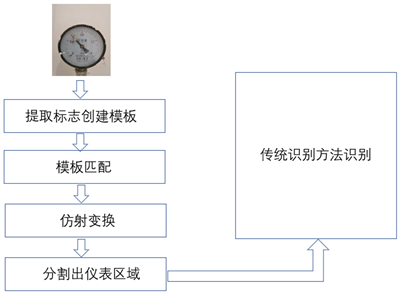Figure 1. Algorithm flowFigure 2. Template area

2.2. 模板匹配

${C}_{fg}=\frac{100}{100}{\sum }_{i=1}^{M}{\sum }_{j=1}^{N}\frac{|{f}_{ij}{\cap }^{\text{​}}{g}_{ij}|}{{T}_{f}+{T}_{g}}$ (1)

2.3. 仿射变换

$HomMat2D=\left[\begin{array}{cc}R& t\\ 00& 1\end{array}\right]=\left[\begin{array}{cc}\begin{array}{cc}1& 0\\ 0& 1\end{array}& t\\ \begin{array}{cc}0& 0\end{array}& 1\end{array}\right]\ast \left[\begin{array}{cc}R& \begin{array}{c}0\\ 0\end{array}\\ 00& 1\end{array}\right]=H\left(t\right)\ast H\left(R\right)$ (2)

$\left(\begin{array}{c}Row2\\ Column2\\ 1\end{array}\right)=HomMat2D\ast \left(\begin{array}{c}Row1\\ Column1\\ 1\end{array}\right)$ (3)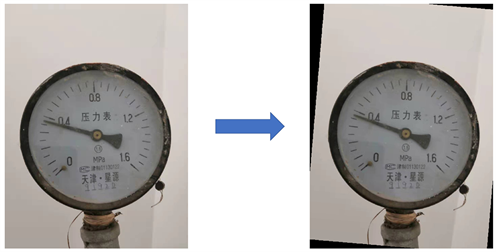Figure 3. Instrument affine transformation and correction

2.4. 分割仪表区域

$R=\mu \left(row1-row2\right)$ (4)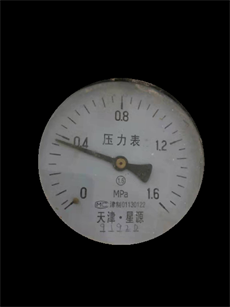Figure 4. Captured image

2.5. 传统识别方法

$Scale=MinValue+\frac{\left(PointerCol-MinCol\right)\ast \left(MaxValue-MinValue\right)}{MaxCol-MinCol}$ (5)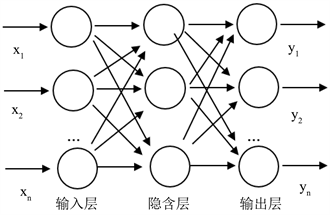Figure 5. BP artificial neural network structure chart

3. 实验结果分析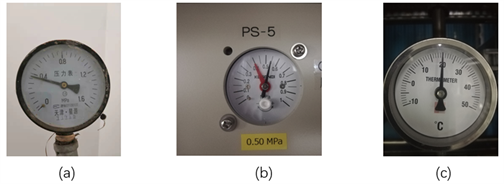Figure 6. Three kinds of pointer metersTable 1. Comparison of recognition results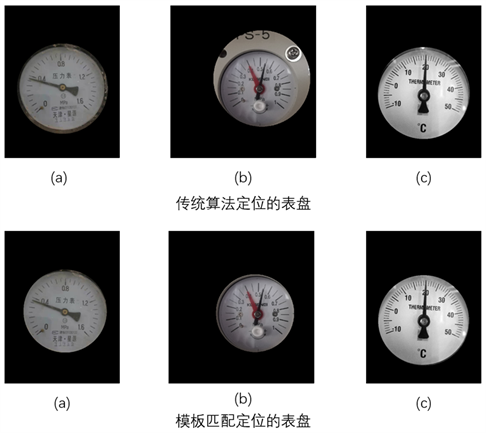Figure 7. Dial positioning in algorithm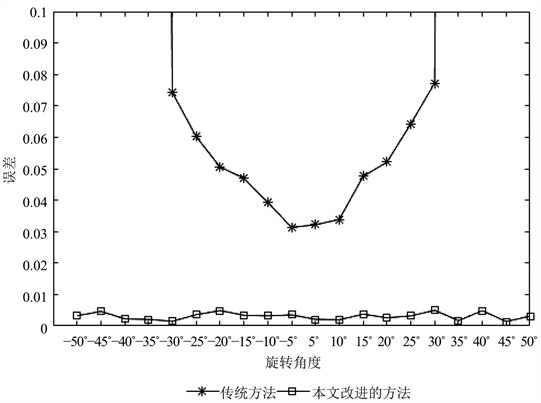Figure 8. Comparison of two algorithms for recognition of rotation angle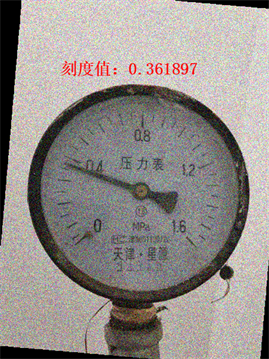Figure 9. Recognition effect after noise treatment

4. 结论

  韩绍超, 徐遵义, 尹中川. 指针式仪表自动读数识别技术的研究现状与发展[J]. 计算机科学, 2018, 45(S1): 54-57.  李勇. 指针式表盘的自动识别技术研究及实现[D]: [硕士学位论文]. 郑州: 郑州大学, 2015.  宋人杰, 赵立亚. Hough变换的改进及其在电厂指针式仪表自动识别中的应用[J]. 东北电力大学学报, 2011, 31(3): 46-50.  晁阳. 基于特征识别的指针式仪表自动识别研究[D]: [硕士学位论文]. 济南: 山东大学, 2008.  段汝娇, 赵伟, 黄松岭, 等. 一种基于改进Hough变换的直线快速检测算法[J]. 仪器仪表学报, 2010, 31(12): 2774-2780.  宋伟, 张文杰, 张家齐, 等. 基于指针区域特征的仪表读数识别算法[J]. 仪器仪表学报, 2014, 35(12): 50-58.  徐鹏, 曾卫明, 石玉虎, 等. 一种指针式油位计读数识别算法[J]. 计算机技术与发展, 2018, 28(9): 189-193.  尹红敏, 葛广英, 肖海俊, 张如如. 基于Halcon的指针式仪表的读数[J]. 现代电子技术, 2016, 39(17): 16-19.  刘见平, 赵丽, 牛树云, 等. 基于形状模板匹配的交通标志污损检测方法研究[J]. 公路交通科技(应用技术版), 2018, 14(12): 278-281.  高聪, 王福龙. 基于模板匹配和局部HOG特征的车牌识别算法[J]. 计算机系统应用, 2017, 26(1): 122-128.  黄剑航. 基于HALCON的圆环区域字符识别实现[J]. 现代计算机(专业版), 2010(7): 58-60.  李锦珑, 张维昭, 马宏锋, 刘馨. 基于HALCON的车牌识别技术研究[J]. 西北师范大学学报: 自然科学版, 2015, 51(6): 54-57.  肖海俊, 葛广英, 姚坤, 等. 基于HALCON的喷码字符识别技术的研究与实现[J]. 现代电子技术, 2015, 38(15): 95-98.  张银苹, 葛广英. 基于HALCON的车牌识别研究[J]. 现代电子技术, 2014, 37(16): 92-95.  张旭兰. 基于BP人工神经网络的车牌字符识别优化算法[J]. 计算机工程与应用, 2012, 48(35): 182-185.